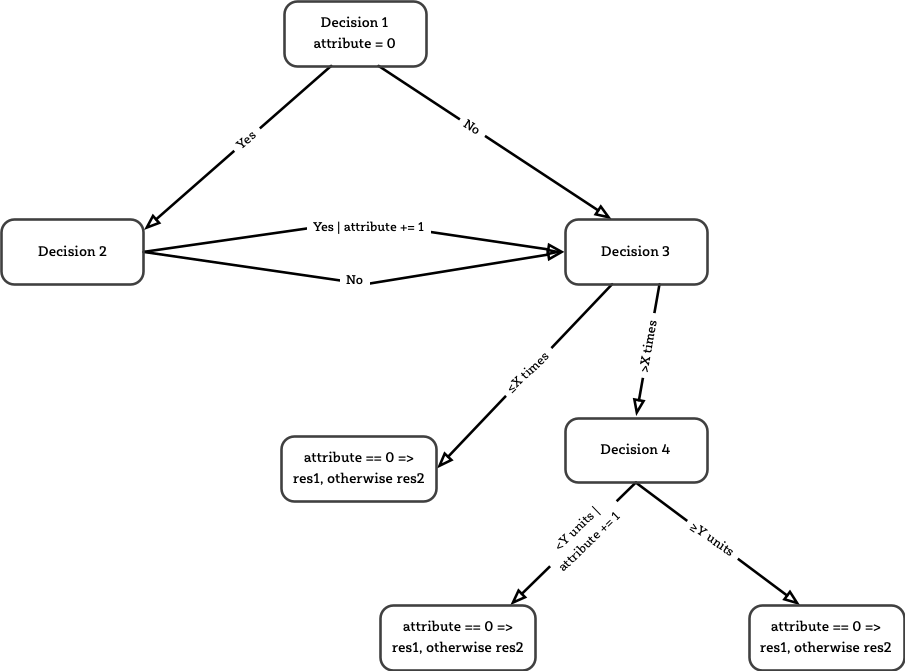# Visualising Paths

In one of my projects we were faced with a high number of execution paths the system could take. While this is normal and, in fact, expected for all systems these days, in this particular project it was obvious even for the users of the system. The software accepts (interactive) input and then demands more information, as needed in the specific context. There were numerous paths through a network of decisions to get to the point where the final result could be presented to the user.

This is basically describing layers of decisions, leading to various outcomes. To get an overview of the decision tree, I created a diagram using an app (OmniGraffle in this case). The result for one real tree (with abstracted labels) is this:Paths trough a decisions tree, updating an attribute of the result

This isn’t trivial, but it doesn’t look too hard. But then, there are 9 different paths thought the graph. The following image shows each path in one colour:

That’s not as simple anymore. Also note, that the attribute is sometimes updated, leading to different result types (res1 for attribute == 0, res2 for all other cases). It was tedious to create these images to help me see the paths — and fiddling the the arrow to make it look reasonably good, was extra work. To deal with more cases I wanted another approach.

I remembered a tool I had used in a similar situation: GraphViz. It’s the combination of a text format (a graph describing language) and programs to turn this into a graphical representation: images.

Here’s the textual representation of the graph above:

```digraph {
size="40";
bgcolor="transparent";

node [fontsize=9, shape=rectangle, style="rounded", color=black];
edge [fontsize=7, fontcolor=red arrowhead=onormal];

D1[ label = "Decision 1\nattribute = 0" ];
D2[ label = "Decision 2" ];
D3[ label = "Decision 3" ];
D4[ label = "Decision 4" ];

R1[ label = "Res 1 | Res 2\ndepending on attribute" ];
R2[ label = "Res 1 | Res 2\ndepending on attribute" ];
R3[ label = "Res 1 | Res 2\ndepending on attribute" ];

D1 -> D2 [label = "Yes"];

D2 -> D3 [label = "Yes\nattribute += 1"];
D2 -> D3 [label = "No"];

D3 -> R1 [label = "≤x times"];
D3 -> D4 [label = ">x times"];

D4 -> R2[label = "<Y units\nattribute += 1"];
D4 -> R3[label = "≤Y units"];
}
```

I find it easy to see which node there are, and how the node connect. Here is the command I used to generate a PNG file:

``dot -Tpng -Gdpi=300 -o decisions.png decisions.dot``

`dot` is one of the layout programs that comes with GraphViz. With `-Ddpi=300` I’ve set the resolution to 300 dpi (to generate a file with a higher resolution than the default). The resulting image file log like this: